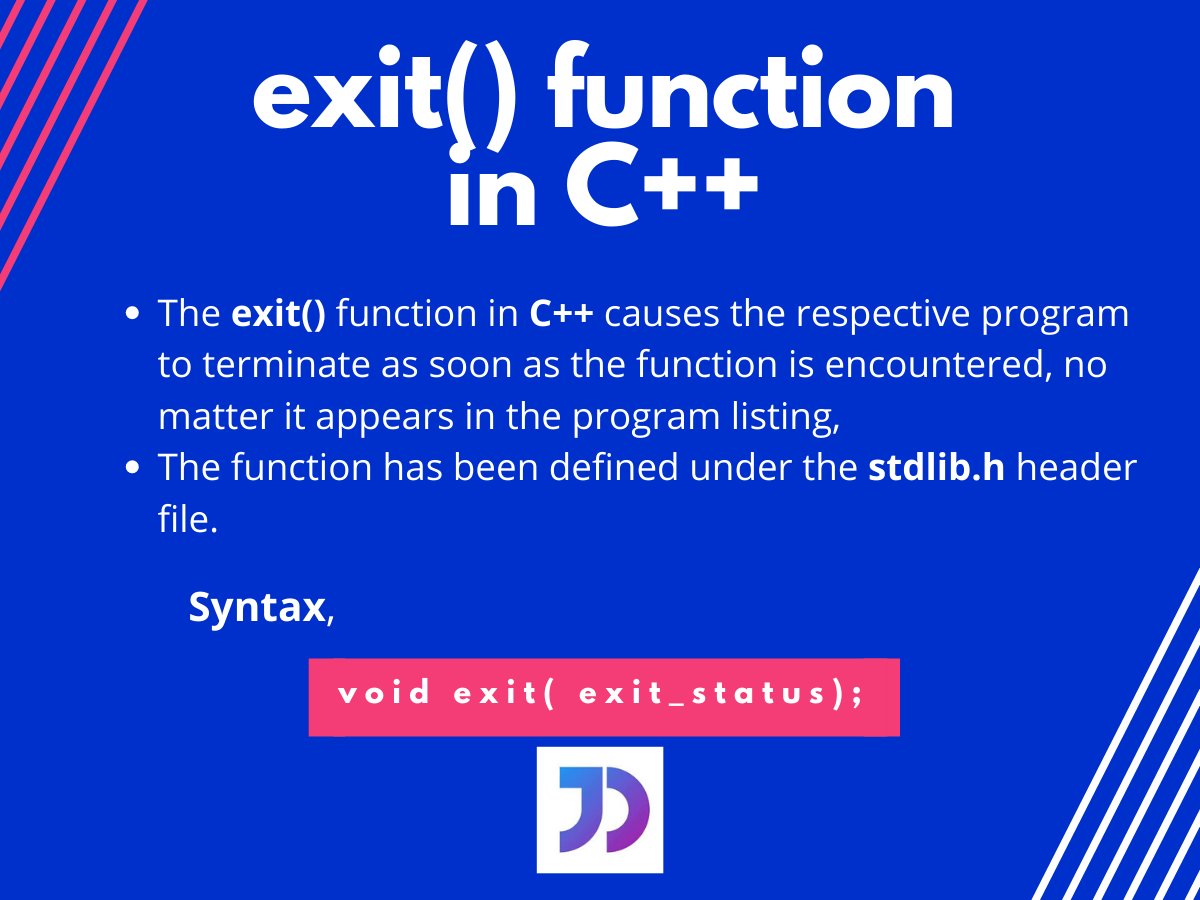# The exit() function in C++

Published on August 3, 2022By Sneh
Developer and author at DigitalOcean.While we believe that this content benefits our community, we have not yet thoroughly reviewed it. If you have any suggestions for improvements, please let us know by clicking the “report an issue“ button at the bottom of the tutorial.

## Introduction

Today we’ll learn about exit() in C++. We know we can break out of loops using the built-in `break` function in C++. Similarly, we can also break out of a whole C++ program using the `exit()` function.

Think of a situation where you are to come to a conclusion in your program. You get the result and conclude something before the whole program has been compiled or evaluated.

How do you terminate the program as soon as you get your required information or result?

The answer to the above question is using the built-in `exit()` function in C++. So let us take a closer look at the function and how it works.

## Definition of the exit() function in C++

Theoretically, the `exit()` function in C++ causes the respective program to terminate as soon as the function is encountered, no matter where it appears in the program listing. The function has been defined under the stdlib.h header file, which must be included while using the exit() function.

## Syntax for the exit() function in C++

The syntax for using the `exit()` function is given below,

``````exit( exit_value );
``````

Here, `exit_value` is the value passed to the Operating system after the successful termination of the program. This value can be tested in batch files where ERROR LEVEL gives us the return value provided by the exit() function. Generally, the value 0 indicates a successful termination and any other number indicates some error.

## Working of the exit() function in C++

Remember, the function `exit()` never returns any value. It terminates the process and performs the regular cleanup for terminating programs.

Also, automatic storage objects are not destroyed by calling this function in C++.

Look at the example below carefully:

``````#include<iostream>
using namespace std;
int main()
{
int i;
cout<<"Enter a non-zero value: ";  //user input
cin>>i;
if(i)    // checks whether the user input is non-zero or not
{
cout<<"Valid input.\n";
}
else
{
cout<<"ERROR!";  //the program exists if the value is 0
exit(0);
}
cout<<"The input was : "<<i;
}
``````

Output:

``````Enter a non-zero value: 0
ERROR!
``````
• Since the user input for the above code provided is zero(0), the else part is executed for the if-else statement. Further where the compiler encounters the `exit()` function and terminates the program.
• Even the print statement below the if-else is not executed since the program has been terminated by the exit() function already.

Now let us look at another example where we try to determine whether a number is prime or not.

## Using the exit() function in C++

The program below illustrates the use of `exit()` function.

``````#include<iostream>
using namespace std;
int main()
{
int i,num;
cout<<"Enter the number : ";
cin>>num;
for(i=2;i<=num/2;i++)
{
if(num%i==0)
{
cout<<"\nNot a prime number!";
exit(0);
}
}
cout<<"\nIt is a prime number!";
return 0;
}
``````

Output:

Further for the above code,

• firstly we took a user input for the number. We need to check whether this number,`num` is prime or not.
• After that, we apply a for loop which works from 2 to n/2. This is because we already know that all numbers are divisible by 1 as well as a number is indivisible by numbers above its half.
• Inside the for loop, we check whether the number is divisible by the loop iterator at that instant. If so, we can directly conclude that the number is not prime and terminate the program using the `exit()` function,
• Else, the loop goes on checking. After the execution of the whole loop structure, we declare the number as a prime one.

## Conclusion

In this tutorial, we discussed the working as well as the usage of the built-in `exit()` function in C++. It is widely used to terminate the execution of a program.

For any questions comment below.